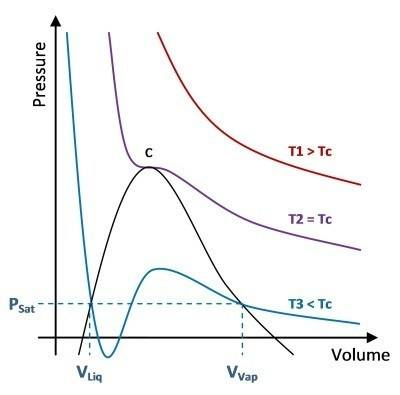# Understanding Cubic Equations of State (Pressure-Volume Relationship)

I am trying to teach myself some chemical thermodynamics, in particular some methods for fluid property evaluation. I am reading an excellent book which has helped a lot ("Introduction to Chemical Engineering Thermodynamics", Smith-Van Ness-Abbott, Ed. 7, ISBN: 007-124708-4).

One thing that the book described, which I am now having some problems with, is the pressure-volume relationship of the cubic equations of state. Figure 3.11 in this book (page 92) shows a nice graph of this relationship with 3 isotherm lines. I have tried to make my own version of this figure (hopefully it is attached ok):The diagram shows that at temperatures less than the critical temperature (e.g. the T3 line) and for low pressures there are 3 vapour solutions (intersections with a horizontal pressure line).

Here is the equation:
$P=\frac{RT}{V-b}-\frac{a}{\left(V+\epsilon b\right)\left(V+\sigma b\right)}$

Where $P$ is pressure, $T$ is temperature and $V$ is molar volume; $a$ is a function of temperature (but is always positive) and $b$, $\epsilon$ and $\sigma$ are positive constants; $R$ is the universal gas constant.

Differentiating this with respect to Volume while keeping T constant (an isotherm line) gives:
$\left(\frac{dP}{dV}\right)_{T}=-\frac{RT}{\left(b-V\right)^{2}}-\frac{a\left(\epsilon b + \sigma b + 2V\right)}{\left(V+\epsilon b\right)^{2}\left(V+\sigma b\right)^{2}}$

Did I do this right? If this differential is correct then I don't see how there can be any extrema (maxima or minima) for any isotherm lines. The 2 terms in the equation would have to cancel each other out in order for the differential to equal zero. As far as I can see both terms will always have the same sign (for positive values of V) meaning they can never cancel out. Is my logic wrong? How does the isotherm T3 get its local minimum and local maximum?

Hope you can help explain this for me.
Ben.

#### Attachments

$\left(\frac{dP}{dV}\right)_{T}=-\frac{RT}{\left(b-V\right)^{2}}-\frac{a\left(\epsilon b + \sigma b + 2V\right)}{\left(V+\epsilon b\right)^{2}\left(V+\sigma b\right)^{2}}$
$\left(\frac{dP}{dV}\right)_{T}=-\frac{RT}{\left(b-V\right)^{2}}+\frac{a\left(\epsilon b + \sigma b + 2V\right)}{\left(V+\epsilon b\right)^{2}\left(V+\sigma b\right)^{2}}$